### Day 75 - Volume: The Disk Method - 12.09.14

• Unit 5/6 Test
• Average = 82%

Bell Ringer Quiz
• Area
1. Find the area between the two functions: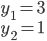from x = 0 to x = 5.

1. 5

2. 10

3. -5

4. 0

5. none of the above

2. Find the area between the two functions: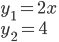from x = 0 to x = 2.

1. -4

2. 4

3. 0

4. 2

5. none of the above

3. Graph each equation. Find the area between the two curves:1. 1/4

2. 1/2

3. 1/12

4. 5/6

5. none of the above

4. Graph each equation. Find the area between the two curves: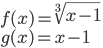1. 1/4

2. 1/2

3. 1/12

4. 5/6

5. none of the above

5. Graph each equation. Find the area bounded by the region: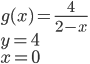1. 4 - ln(2)

2. 4 - 4ln(2)

3. 4

4. 0

5. none of the above

Review
• Prerequisites
• Integration
• Area Between Two Curves

Lesson
• Volume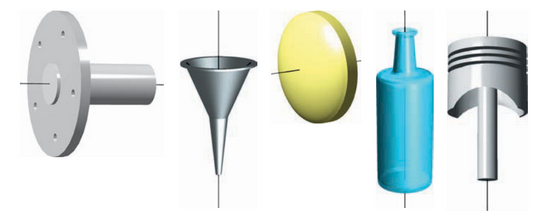• The Disk Method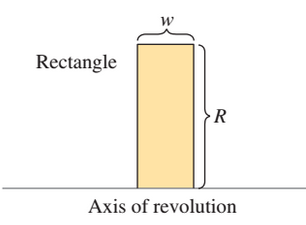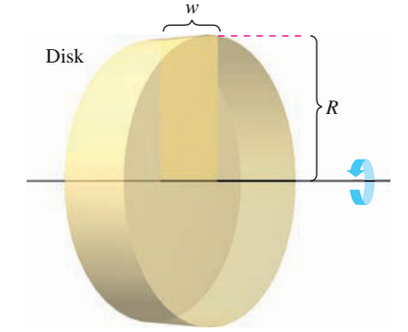• The Washer Method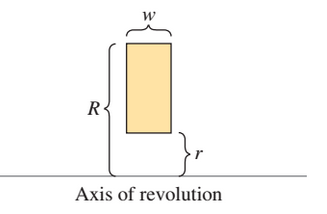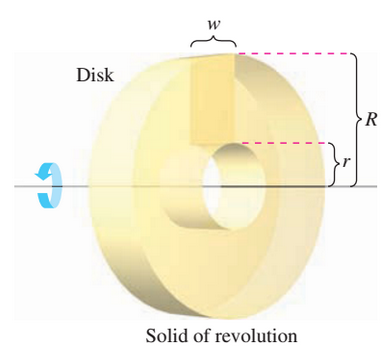Exit Ticket
• Posted on the board at the end of the block.
Lesson Objective(s)
• How can definite integrals be used to find the volume of a solid of revolution?

#### In-Class Help Requests

Standard(s)
• APC.9

• Apply formulas to find derivatives.

• Includes:

• derivatives of algebraic, trigonometric, exponential, logarithmic, and inverse trigonometric functions

• derivations of sums, products, quotients, inverses, and composites (chain rule) of elementary functions

• derivatives of implicitly defined functions

• higher order derivatives of algebraic, trigonometric, exponential, and logarithmic, functions

Math
ematical Practice(s)
• #1 - Make sense of problems and persevere in solving them
• #2 - Reason abstractly and quantitatively
• #5 - Use appropriate tools strategically
• #6 - Attend to precision
• #8 - Look for and express regularity in repeated reasoning

Past Checkpoints

Equations

Area Bounded by Two Functions

Disk Method

Washer Method